Related Articles
String manipulations in Pandas DataFrame
• Last Updated : 01 Aug, 2020

String manipulation is the process of changing, parsing, splicing, pasting, or analyzing strings. As we know that sometimes, data in the string is not suitable for manipulating the analysis or get a description of the data. But Python is known for its ability to manipulate strings. So, by extending it here we will get to know how Pandas provides us the ways to manipulate to modify and process string data-frame using some builtin functions. Pandas library have some of the builtin functions which is often used to String Data-Frame Manipulations

First of all, we will know ways to create a string data-frame using pandas:

## Python3

 `# Importing the necessary libraries``import` `pandas as pd``import` `numpy as np`` ` `# df stands for dataframe``df ``=` `pd.Series([``'Gulshan'``, ``'Shashank'``, ``'Bablu'``,``                ``'Abhishek'``, ``'Anand'``, np.nan, ``'Pratap'``])`` ` `print``(df)`

Output: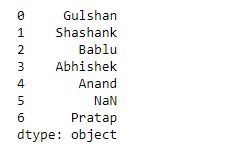Let’s change the type of the above-created dataframe to string type. There can be various methods to do the same. Let’s have a look at them in the below examples.

Example 1: We can change the dtype after the creation of data-frame:

## Python3

 `# we can change the dtype after``# creation of dataframe``print``(df.astype(``'string'``))`

Output: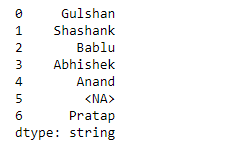Example 2: Creating the dataframe as dtype = ‘string’:

## Python3

 `# now creating the dataframe as dtype = 'string'``import` `pandas as pd``import` `numpy as np`` ` `df ``=` `pd.Series([``'Gulshan'``, ``'Shashank'``, ``'Bablu'``, ``'Abhishek'``,``                ``'Anand'``, np.nan, ``'Pratap'``], dtype``=``'string'``)`` ` `print``(df)`

Output:Example 3: Creating the dataframe as dtype = pd.StringDtype():

## Python3

 `# now creating the dataframe as dtype = pd.StringDtype()``import` `pandas as pd``import` `numpy as np`` ` `df ``=` `pd.Series([``'Gulshan'``, ``'Shashank'``, ``'Bablu'``, ``'Abhishek'``,``                ``'Anand'``, np.nan, ``'Pratap'``], dtype``=``pd.StringDtype())`` ` `print``(df)`

Output:## String Manipulations in Pandas

Now, we see the string manipulations inside a pandas data frame, so first, create a data frame and manipulate all string operations on this single data frame below, so that everyone can get to know about it easily.

Example:

## Python3

 `# python script for create a dataframe``# for string manipulations``import` `pandas as pd``import` `numpy as np`` ` `df ``=` `pd.Series([``'night_fury1'``, ``'Is  '``, ``'Geeks, forgeeks'``,``                ``'100'``, np.nan, ``'  Contributor '``])``df`

Output: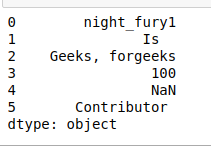Let’s have a look at various methods provided by this library for string manipulations.

• lower(): Converts all uppercase characters in strings in the DataFrame to lower case and returns the lowercase strings in the result.

## Python3

 `# lower()``print``(df.``str``.lower())`
```0        night_fury1
1               is
2    geeks, forgeeks
3                100
4                NaN
5       contributor
dtype: object
```
• upper(): Converts all lowercase characters in strings in the DataFrame to upper case and returns the uppercase strings in result.

## Python3

 `#upper()``print``(df.``str``.upper())`

Output: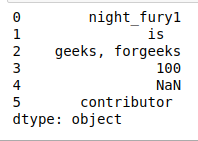• strip(): If there are spaces at the beginning or end of a string, we should trim the strings to eliminate spaces using strip() or remove the extra spaces contained by a string in DataFrame.

## Python3

 `# strip()``print``(df)``print``(``'\nAfter using the strip:'``)``print``(df.``str``.strip())`

Output: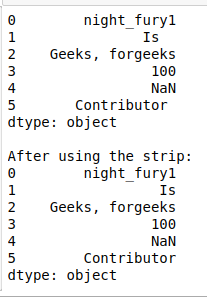• split(‘ ‘): Splits each string with the given pattern. Strings are split and the new elements after the performed split operation, are stored in a list.

## Python3

 `# split(pattern)``print``(df)``print``(``'\nAfter using the strip:'``)``print``(df.``str``.split(``','``))`` ` `# now we can use [] or get() to fetch ``# the index values``print``(``'\nusing []:'``)``print``(df.``str``.split(``','``).``str``[``0``])`` ` `print``(``'\nusing get():'``)``print``(df.``str``.split(``','``).``str``.get(``1``))`

Output: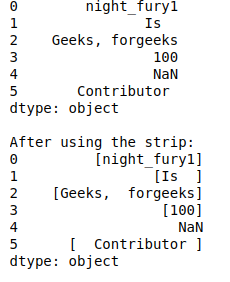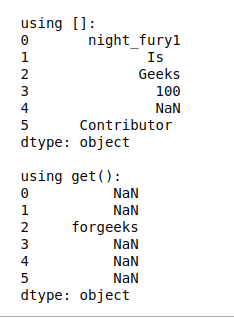• len(): With the help of len() we can compute the length of each string in DataFrame & if there is empty data in DataFrame, it returns NaN.

## Python3

 `# len()``print``(``"length of the dataframe: "``, ``len``(df))``print``(``"length of each value of dataframe:"``)``print``(df.``str``.``len``())`

Output: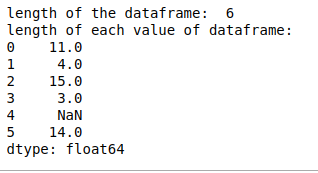• cat(sep=’ ‘): It concatenates the data-frame index elements or each string in DataFrame with given separator.

## Python3

 `# cat(sep=pattern)``print``(df)`` ` `print``(``"\nafter using cat:"``)``print``(df.``str``.cat(sep``=``'_'``))`` ` `print``(``"\nworking with NaN using cat:"``)``print``(df.``str``.cat(sep``=``'_'``, na_rep``=``'#'``))`

Output: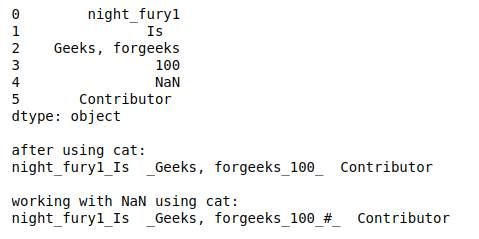• get_dummies(): It returns the DataFrame with One-Hot Encoded values like we can see that it returns boolean value 1 if it exists in relative index or 0 if not exists.

## Python3

 `# get_dummies()``print``(df.``str``.get_dummies())`

Output: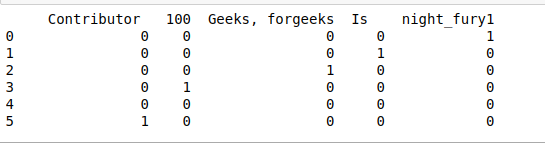• startswith(pattern): It returns true if the element or string in the DataFrame Index starts with the pattern.

## Python3

 `# startswith(pattern)``print``(df.``str``.startswith(``'G'``))`

Output: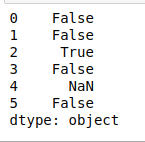• endswith(pattern): It returns true if the element or string in the DataFrame Index ends with the pattern.

## Python3

 `# endswith(pattern)``print``(df.``str``.endswith(``'1'``))`

Output: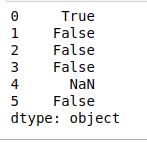• replace(a,b): It replaces the value a with the value b like below in example ‘Geeks’ is being replaced by ‘Gulshan’.

## Python3

 `# replace(a,b)``print``(df)``print``(``"\nAfter using replace:"``)``print``(df.``str``.replace(``'Geeks'``, ``'Gulshan'``))`

Output: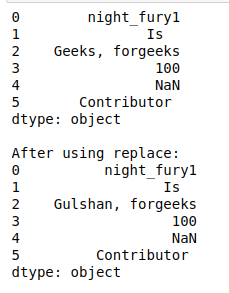• repeat(value): It repeats each element with a given number of times like below in example, there are two appearances of each string in DataFrame.

## Python3

 `# repeat(value)``print``(df.``str``.repeat(``2``))`

Output: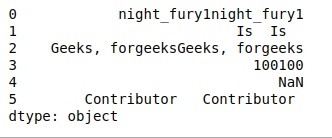• count(pattern): It returns the count of the appearance of pattern in each element in Data-Frame like below in example it counts ‘n’ in each string of DataFrame and returns the total counts of ‘n’ in each string.

## Python3

 `# count(pattern)``print``(df.``str``.count(``'n'``))`

Output: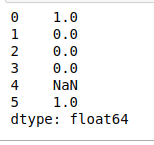• find(pattern): It returns the first position of the first occurrence of the pattern. We can see in the example below, that it returns the index value of appearance of character ‘n’ in each string throughout the DataFrame.

## Python3

 `# find(pattern)``# in result '-1' indicates there is no``# value matching with given pattern in ``# particular row``print``(df.``str``.find(``'n'``))`

Output: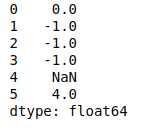• findall(pattern): It returns a list of all occurrences of the pattern. As we can see in below, there is a returned list consisting n as it appears only once in the string.

## Python3

 `# findall(pattern)``# in result [] indicates null list as ``# there is no value matching with given``# pattern in particular row``print``(df.``str``.findall(``'n'``))`

Output: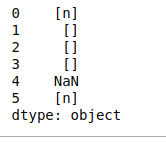• islower(): It checks whether all characters in each string in the Index of the Data-Frame in lower case or not, and returns a Boolean value.

## Python3

 `# islower()``print``(df.``str``.islower())`

Output: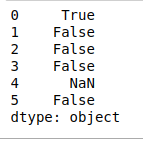• isupper(): It checks whether all characters in each string in the Index of the Data-Frame in upper case or not, and returns a Boolean value.

## Python3

 `# isupper()``print``(df.``str``.isupper()) `

Output: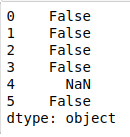• isnumeric(): It checks whether all characters in each string in the Index of the Data-Frame are numeric or not, and returns a Boolean value.

## Python3

 `# isnumeric()``print``(df.``str``.isnumeric())`

Output: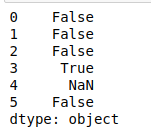• swapcase(): It swaps the case lower to upper and vice-versa. Like in the example below, it converts all uppercase characters in each string into lowercase and vice-versa (lowercase -> uppercase).

## Python3

 `# swapcase()``print``(df.``str``.swapcase())`

Output: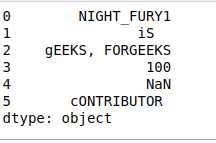Attention geek! Strengthen your foundations with the Python Programming Foundation Course and learn the basics.

To begin with, your interview preparations Enhance your Data Structures concepts with the Python DS Course.

My Personal Notes arrow_drop_up